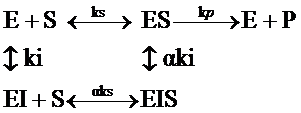# Enzyme Inhibition

Enzymes are proteins that speed up the rate of a reaction by providing an alternate route to overcoming the activation energy. The graph below shows the path of a reaction both with and without the presence of an enzyme.

## Introduction

The black line indicates the path a reaction would take in the absence of an enzyme while the yellow line indicates the path a reaction would take with an enzyme.The general reaction of an enzyme is $$E+S \rightleftharpoons ES \rightleftharpoons E + P$$ and the velocity of the reaction is given by the Michaelis-Menten equation:

$v=\dfrac{v_{max} [S]}{k_m +[S]}$

I is the concentration of the inhibitor and $$K_i$$ is the dissociation constant of the $$E_I$$ complex. A Lineweaver-Burke plot of 1/V vs. 1/S shows us how the Km and the $$V_{max}$$ change as inhibitor concentration changes. The red line corresponds to the reaction with no inhibitor present while the black lines are with various inhibitor concentration. The black arrow shows an increasing inhibitor concentration. We see from the graph that a competitive inhibitor changes the apparent value of Km but does not affect the Vmax.Figure 2: A Lineweaver-Burke plot of $$1/V$$ vs. $$1/S$$

## Noncompetitive Inhibition

In noncompetitive inhibition, the inhibitor binds to the enzyme at a location other than the active site in such a way that the inhibitor and substrate can simultaneously be attached to the enzyme. The substrate and the inhibitor have no effect on the binding of the other and can bind and unbind the enzyme in either order. The inhibitor and the substrate are not binding to the same site. The general reaction for this is

$E+S \rightleftharpoons ES \rightleftharpoons E + P$

except at the same time other processes may occur:

$E+I \rightleftharpoons EI$

$ES+I \rightleftharpoons EIS$

and

$EI+S \rightleftharpoons EIS$.

The Michaelis-Menten equation for this becomes:

$v=\dfrac{v_{max}[S]}{k_m \left(1+\dfrac{[I]}{k_i} \right)+[S] \left(1+\dfrac{[I]}{k_i} \right)}$

In uncompetitive inhibition, the inhibitor binds to the enzyme only after the substrate has bound. The general reaction for this is

$E+S \rightleftharpoons ES \rightleftharpoons E + P$

except at the same time ES+I <-> ESI. The Michaelis Menten equation for this becomes:

$v=\dfrac{v_{max} [S]}{k_m +[S] \left(1+\dfrac{[I]}{k_i} \right)}$

where $$I$$ is the concentration of uncompetitive inhibitor and $$K_i$$ is the dissociation constant of the $$ESI$$ complex.

The Lineweaver-Burke plot below shows that for uncompetitive inhibition both the apparent $$K_M$$ and apparent $$v_{max}$$ decrease with increase in inhibitor concentration.Figure : Lineweaver-Burke

## Mixed Type Inhibition

Mixed type inhibition is similar to noncompetitive inhibition except that binding of the substrate or the inhibitor affect the enzyme’s binding affinity for the other. The change in binding affinity is included in the chemical equation by the term ki. For mixed type inhibition ki>1, which means that binding affinity for the substrate is decreased when the inhibitor is present.Note that it is possible to completely deactivate the enzye activity by saturating it with I because the EIS complex does not form product.The velocity equation for mixed type is:

$v=\dfrac{v_{max}[S]}{k_s \left(1+\dfrac{[I]}{k_i} \right)+[S] \left(1+\dfrac{[I]}{\alpha k_i} \right)}$As usual, the basic velocity graph is not very useful, so we take the double reciprocal of the velocity equation. The Lineweaver-Burke equation for mixed type is:Due to the ? factor, we cannot solve for all of the kinetic constants from the Lineweaver-Burke plot alone. To solve this problem, we make re-plots of the slope and the y-intercept at varying concentrations of I.p style="text-align: justify;">Determining the kinetic constants

• Ks: Can be found from the original double reciprocal plot ([I]=0 line)
• vmax: Can be found in the original double reciprocal plot ([I]=0 line)
• Ki: Can be found from the slope of the slope re-plot
• ?: Can be found from the slope of the y-intercept re-plot

## Partial Mixed Type Inhibition

Partial mixed type inhibition is similar to mixed type inhibition except that the inhibited, substrate-bound EIS complex maintains a reduced level of catalytic activity. This means that an inhibitor can never stop the reaction, even at saturating concentration. Partial mixed type still has the ? factor that raises ks, but it also has a ? factor that decreases vmax. At saturating [I], ks approaches ?ks and vmax approaches ?vmax.

The general and velocity equations are:Again, we can take the double reciprocal and look at the line with no inhibitor to determine ks and vmax.Similar to mixed type inhibition, re-plots of the slope and intercept are needed to determine ?, ?, and Ki. However, in this case, the re-plots are curved, so a few extra manipulations are necessary to get information from these graphs.First, the y-intercept is subtracted from the equation to force the curves to go through the origin. These equations are called ?slope and ?y-intercept, and the double reciprocals of these ?plots can be taken to solve for ?, ?, and Ki.\Determining the kinetic constants

• Ks: Can be found in the original double reciprocal plot ([I]=0 line)
• vmax: Can be found in the original double reciprocal plot ([I]=0 line)
• ?: Can be found from the y-intercept of the inverse y-intercept graph.
• ?: After finding ?, ? can be found from the y-intercept of the inverse slope graph.
• Ki: After finding ? and ?, Ki can be determined from either ?plot.

## Sources

1. Atkins, Peter, and Julio De Paula. Physical Chemistry for the Life Sciences. New York: W.H. Freeman and Company, 2006.
2. Segel, Irwin. Enzyme Kinetics. John Wiley & Sons, Inc., 1993.
3. Garrett, Reginald H., and Charles M. Grisham. Biochemistry. Belmont: Thomson Brooks/Cole, 2005.

## Contributors

• Peter Abboud, Julie Choe, Kristopher Restel